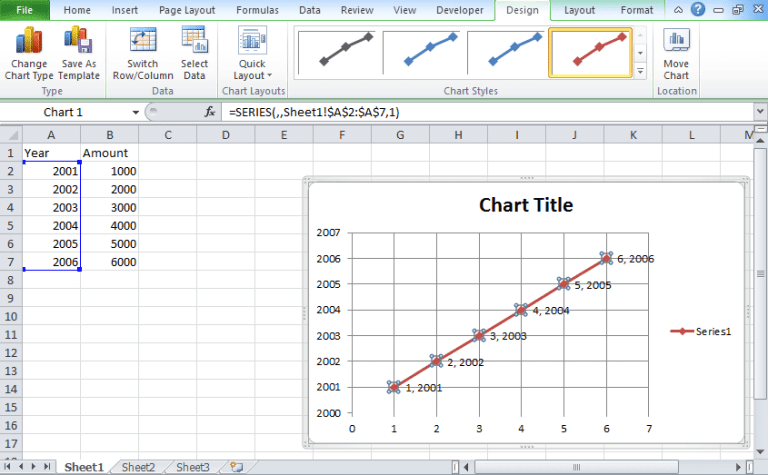To advertise with us contact on Whatsapp: +923041280395 For guest post email at: [email protected]

# Regression Analysis in Excel [Linear Regression]Whenever we want to estimate the relationship between variables then we think of using regression analysis. There are different types of regressions that you can perform. Here we are going to tell you how to use regression analysis in excel to understand which of the independent variable are related to which of the dependent variable.

## Regression Analysis in Excel

You can get a visual regression analysis using the scatter plotting technique. To plot graph, you need to use the regression tool that is provided by the Data Analysis tool. The Data Analysis tab comes using an add-in function.

### Simple Regression Analysis

In the simple regression analysis, you will find a single variable “X” for every dependent variable “Y”.

### Multiple Regression Analysis

In the multiple regression analysis, you will find a significant relationship between the sets of variables. Using this you can find the trends among those data sets.

## Regression Equation Formula

The regression equation is also called a slope formula.

Y=a+bX where Y is said to be a dependent variable, X is the independent variable, a is the intercept of Y-axis and b is the slope of the line.

### How to create regression equation in Excel?

• You need to add scatterplot graph in your excel sheet using the data.
• Choose the x-axis i.e, horizontal line and y-axis i.e, a vertical line.
• Click Ok.
• After this, you will find a scatterplot.
• Click right button on any of the dots.
• Choose the required trendline option. Here we have selected a linear one.
• Select the display equation on chart and display the R-squared value on chart.
• After doing so, we will find a scatterplot with the trendline equation.
• On the graph, you will find the regression formula.
• Here the formula is Y=X+2000.

### How to use regression analysis in Excel?

To perform regression analysis, you need to add data analysis tab. The data analysis tab is added using Add-Ins and Analysis ToolPak list.

• After doing so, go to the data tab.
• In the data tab, you will find data analysis icon.
• Click on the data analysis.
• You will get a pop-up menu.
• In the pop-up menu, choose regression.
• After choosing regression, you will get another pop-up where you need to mention the range of Y and range of X
• If the value of the dependent variable is equal to zero when the independent value equals to zero then select Constant is Zero option else not.
• Choose the data that you want. You can choose the normal probability plot checkbox to add-on residuals and to get regression analysis result.
• Press ok.
• You will find three portion regression analysis with stacked visual plots of data.

This is how you can perform single and multiple regression analysis in excel. Check the process to create a scatterplot. If you have any doubt then you can mention below in the comment box and one of our team members will get back with the answer. You can also check other graphs like Pareto chart, histogram chart, and other charts in our excel tutorial.